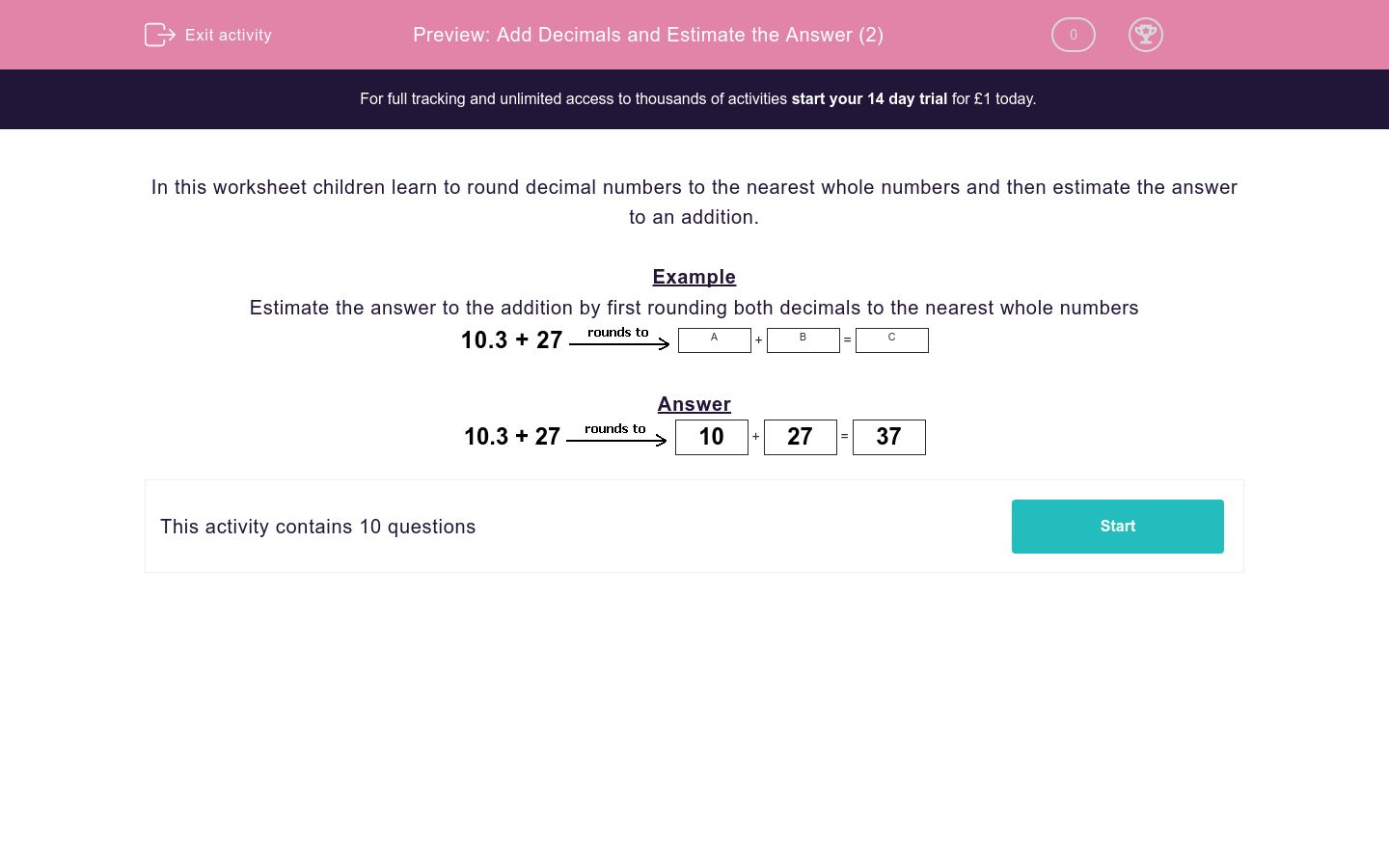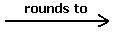### Affordable pricing from £10/month

In this worksheet, students round decimal numbers to the nearest whole numbers in order to estimate the answer to an addition.Key stage:  KS 2

Curriculum topic:   Maths and Numerical Reasoning

Curriculum subtopic:   Decimals

Difficulty level:### QUESTION 1 of 10

In this worksheet children learn to round decimal numbers to the nearest whole numbers and then estimate the answer to an addition.

Example

Estimate the answer to the addition by first rounding both decimals to the nearest whole numbers

10.3 27A
+
 B
=
 C

10.3 2710
+
 27
=
 37

Estimate the answer by rounding the numbers to the nearest 100.

304 + 893A
+
 B
=
 C

Remember to put your answers in the same sequence as the question!

For example, A would be 304 to the nearest 100 and B would be 893...

## Column B

C
300
A
1200
B
900

Estimate the answer to the addition by first rounding both decimals to the nearest whole numbers.

27.8 + 15.4A
+
 B
=
 C

Remember to put your answers in the same sequence as the question!

For example, A would be 27.8 to the nearest whole number and B would be 15.4...

## Column B

A
15
B
28
C
43

Estimate the answer to the addition by first rounding both decimals to the nearest whole numbers.

11.8 + 10.5A
+
 B
=
 C

Remember to put your answers in the same sequence as the question!

For example, A would be 11.8 to the nearest whole number and B would be 10.5...

## Column B

A
23
B
11
C
12

Estimate the answer to the addition by first rounding both decimals to the nearest whole numbers.

68.6 + 58.7A
+
 B
=
 C

Remember to put your answers in the same sequence as the question!

## Column B

A
69
B
59
C
128

Estimate the answer to the addition by first rounding both decimals to the nearest whole numbers.

59.7 + 56.4A
+
 B
=
 C

Remember to put your answers in the same sequence as the question!

## Column B

A
56
B
116
C
60

Estimate the answer to the addition by first rounding both decimals to the nearest whole numbers.

57.6 + 47.8A
+
 B
=
 C

Remember to put your answers in the same sequence as the question!

## Column B

A
48
B
106
C
58

Estimate the answer to the addition by first rounding both decimals to the nearest whole numbers.

79.1 + 22.1A
+
 B
=
 C

Remember to put your answers in the same sequence as the question!

## Column B

A
101
B
22
C
79

Estimate the answer to the addition by first rounding both decimals to the nearest whole numbers.

26.7 + 22.6A
+
 B
=
 C

Remember to put your answers in the same sequence as the question!

## Column B

A
27
B
23
C
50

Estimate the answer to the addition by first rounding both decimals to the nearest whole numbers.

46.9 + 32.5A
+
 B
=
 C

Remember to put your answers in the same sequence as the question!

## Column B

A
47
B
80
C
33

Estimate the answer to the addition by first rounding both decimals to the nearest whole numbers.

94.9 + 31A
+
 B
=
 C

Remember to put your answers in the same sequence as the question!

## Column B

A
95
B
31
C
126
• Question 1

Estimate the answer by rounding the numbers to the nearest 100.

304 + 893A
+
 B
=
 C

Remember to put your answers in the same sequence as the question!

For example, A would be 304 to the nearest 100 and B would be 893...

## Column B

C
1200
A
300
B
900
• Question 2

Estimate the answer to the addition by first rounding both decimals to the nearest whole numbers.

27.8 + 15.4A
+
 B
=
 C

Remember to put your answers in the same sequence as the question!

For example, A would be 27.8 to the nearest whole number and B would be 15.4...

## Column B

A
28
B
15
C
43
• Question 3

Estimate the answer to the addition by first rounding both decimals to the nearest whole numbers.

11.8 + 10.5A
+
 B
=
 C

Remember to put your answers in the same sequence as the question!

For example, A would be 11.8 to the nearest whole number and B would be 10.5...

## Column B

A
12
B
11
C
23
• Question 4

Estimate the answer to the addition by first rounding both decimals to the nearest whole numbers.

68.6 + 58.7A
+
 B
=
 C

Remember to put your answers in the same sequence as the question!

## Column B

A
69
B
59
C
128
• Question 5

Estimate the answer to the addition by first rounding both decimals to the nearest whole numbers.

59.7 + 56.4A
+
 B
=
 C

Remember to put your answers in the same sequence as the question!

## Column B

A
60
B
56
C
116
• Question 6

Estimate the answer to the addition by first rounding both decimals to the nearest whole numbers.

57.6 + 47.8A
+
 B
=
 C

Remember to put your answers in the same sequence as the question!

## Column B

A
58
B
48
C
106
• Question 7

Estimate the answer to the addition by first rounding both decimals to the nearest whole numbers.

79.1 + 22.1A
+
 B
=
 C

Remember to put your answers in the same sequence as the question!

## Column B

A
79
B
22
C
101
• Question 8

Estimate the answer to the addition by first rounding both decimals to the nearest whole numbers.

26.7 + 22.6A
+
 B
=
 C

Remember to put your answers in the same sequence as the question!

## Column B

A
27
B
23
C
50
• Question 9

Estimate the answer to the addition by first rounding both decimals to the nearest whole numbers.

46.9 + 32.5A
+
 B
=
 C

Remember to put your answers in the same sequence as the question!

## Column B

A
47
B
33
C
80
• Question 10

Estimate the answer to the addition by first rounding both decimals to the nearest whole numbers.

94.9 + 31A
+
 B
=
 C

Remember to put your answers in the same sequence as the question!

## Column B

A
95
B
31
C
126
---- OR ----

Sign up for a £1 trial so you can track and measure your child's progress on this activity.

### What is EdPlace?

We're your National Curriculum aligned online education content provider helping each child succeed in English, maths and science from year 1 to GCSE. With an EdPlace account you’ll be able to track and measure progress, helping each child achieve their best. We build confidence and attainment by personalising each child’s learning at a level that suits them.

Get started